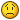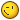# Rotated Text collision

## Recommended Posts

Hi everyone!

I have several Pixi.Text objects floating in my Pixi container.

``````var style = {
font:"22px Verdana",
fill:getRandomColor()
};

var text = new PIXI.Text("My text", style);

text.anchor.set(0.5, 0.5);

text.dx = 0.1;
text.dy = 0.1;``````

Each Text object is rotating from -90 degrees to + 90 degrees.

When collision happens with Text object and container, Text acts like a ping-pong ball changing its direction.

Currently I'm using this code to check if text object is < or > of my pixi container width:

``````if(text.x < 0 || text.x > \$("#pixi-container").width())
text.dx = -text.dx;
if(text.y < 0 || text.y > \$("#pixi-container").height())
text.dy = -text.dy;

text.x += text.dx;
text.y += text.dy;``````

Collision happens when a center of text object reaches container border.

I need collision detection when ends of text object touches the container border.

##### Share on other sites

I tried this but it doesn't calculates collision properly because of rotating angle:

``````if((text.x - (text.width / 2)) < 0 || (text.x + (text.width / 2)) > \$("#pixi-container").width())
text.dx = -text.dx;
if((text.y - (text.width / 2)) < 0 || (text.y + (text.width / 2)) > \$("#pixi-container").height())
text.dy = -text.dy;``````

##### Share on other sites

Another try to calculate max length X and max length Y regarding rotating angle:

``````var lengthX = (0.5 * text.width * Math.abs(Math.cos(text.rotation))) + (0.5 * text.height * Math.abs(Math.cos(text.rotation)));
var lengthY = (0.5 * text.width * Math.abs(Math.sin(text.rotation))) + (0.5 * text.height * Math.abs(Math.sin(text.rotation)));

if((text.x - lengthX) < 0 || (text.x + lengthX) > \$("#pixi-container").width())
text.dx = -text.dx;
if((text.y - lengthY) < 0 || (text.y + lengthY) > \$("#pixi-container").height())
text.dy = -text.dy;``````

I'm not mathematician and this equation is help of my friend but there is an error somewhere.

Does anyone can help me solve this.

Edited by NavCore
Removed conversion from radians to degrees to make it work
##### Share on other sites

This should work, it looks like you copy and pasted the horizontal check and forgot to change text.width to text.height. Otherwise the logic seems fine.

``````if(text.x - text.width / 2 < 0 || text.x + text.width / 2 > \$("#pixi-container").width()) {
text.dx = -text.dx;
}
if(text.y - text.height / 2 < 0 || text.y + text.height / 2 > \$("#pixi-container").height()) {
text.dy = -text.dy;
}``````

P.S. It's probably better practice to use renderer.width and renderer.height instead of the HTML canvas's width and height.

##### Share on other sites

24 minutes ago, Taz said:

This should work, it looks like you copy and pasted the horizontal check and forget to change text.width to text.height. Otherwise it should work.

```
```if((text.x - (text.width / 2)) < 0 || (text.x + (text.width / 2)) > \$("#pixi-container").width()) {
text.dx = -text.dx;
}
if((text.y - (text.height / 2)) < 0 || (text.y + (text.height / 2)) > \$("#pixi-container").height()) {
text.dy = -text.dy;
}```
```

That is not a solution. Imagine that text is rotated -90 or +90 degrees and it is comparing text.y.
In that case it should be compared with text.width / 2 because that is a maximum.

It's all about mathematics calculaction, something similar to my previous post.
LengthX and lengthY should be calculated in any moment to measure a precise collision regarding text object rotation.

##### Share on other sites

``````var bounds = text.getBounds();

if(bounds.x < 0 || bounds.x + bounds.width > \$("#pixi-container").width()) {
text.dx = -text.dx;
}
if(bounds.y < 0 || bounds.y + bounds.height > \$("#pixi-container").height()) {
text.dy = -text.dy;
}``````

Or like this using renderer dimensions is better I think:

``````var bounds = text.getBounds();

if(bounds.x < 0 || bounds.x + bounds.width > renderer.width) {
text.dx = -text.dx;
}
if(bounds.y < 0 || bounds.y + bounds.height > renderer.height) {
text.dy = -text.dy;
}``````

##### Share on other sites

Mate, when I saw this bounds methods I thought Taz you made my day.

But I just tried this bound-method and it results in objects goes out of boundaries.

``````var bounds = text.getBounds();

if(bounds.x < 0 || bounds.x + bounds.width > renderer.width)
text.dx = -text.dx;
if(bounds.y < 0 || bounds.y + bounds.height > renderer.height)
text.dy = -text.dy;

text.x += text.dx;
text.y += text.dy;``````

Take a look at attached image.I also tried to compare text.x instead bounds.x as well as text.y instead bounds.y but it's not working.##### Share on other sites

Okay, I need to do this with sprites to detect laser collisions with space ships in my game eventually, so I'm gonna make a CodePen and see if I can get it working. I'll test with sprites and with text too while I'm at it. Will report back and post the CodePen when I have some results.

##### Share on other sites

Great, thanks in advanced !!##### Share on other sites

Hey, this CodePen seems to be working using getBounds like above post. I tried with various angles and the text stays within the window, bouncing off the edges.

##### Share on other sites

45 minutes ago, Taz said:

Hey, this CodePen seems to be working using getBounds like above post. I tried with various angles and the text stays within the window, bouncing off the edges.

Thanks for the CodePen example.

You used fixed rotation angle all the time.
That way it works also in my example.

But when the rotation is changing all the time, then it doesn't calculate text bounds well in my code.

I will expand CodePen example and make dynamic rotation to show the problem.

##### Share on other sites

Here is a rotation example that doesn't calculate boundaries properly:

##### Share on other sites

I think what's happening is that when the angle rotates when the text is close to the edge, then the text becomes already over the edge, before the collision check even happens. Then the collision check changes the direction, but it takes a few frames for it to move back into the window. So the collision check needs to do more than just change the velocity. It needs to actually change the position so that the text is exactly at the edge of the window. The angle and text dimensions can be used to figure out exactly how far to move the text. Give me a few minutes to contemplate the math and I'll maybe have something##### Share on other sites

I figured out why is this happening =)

Only needed to add this line inside matching condition: text.rotationVal = -text.rotationVal; to change the rotation direction because rotation tried to break through the boundaries.

The working code is:

``````if(bounds.x < 0 || bounds.x + bounds.width > renderer.width) {
text.dx = -text.dx;
text.rotationVal = -text.rotationVal;
}

if(bounds.y < 0 || bounds.y + bounds.height > renderer.height) {
text.dy = -text.dy;
text.rotationVal = -text.rotationVal;
}``````

CodePen example updated:

Thank you very much Taz for your help!

Have a nice day !!

:: cheers ::

##### Share on other sites

Okay this is working, no math required after all, thank goodness!``````  var bounds = text.getBounds();
if(bounds.x < 0) {
text.x -= bounds.x;

text.dx = -text.dx;
}
else if (bounds.x + bounds.width > renderer.width) {
text.x -= bounds.x + bounds.width -renderer.width;

text.dx = -text.dx;
}

if(bounds.y < 0) {
text.y -= bounds.y;

text.dy = -text.dy;
}
else if(bounds.y + bounds.height > renderer.height) {
text.dy = -text.dy;

text.y -= bounds.y + bounds.height -renderer.height;
}``````

##### Share on other sites

LOL, we fixed at same timeYours looks simpler, more concise thou - I think it's the winner##### Share on other sites

No problem, was a fun distraction and I learned a thing or two along the wayCheers

##### Share on other sites

Yeah, me toThis bounds thing is very helpful - thanks again for the tips##### Share on other sites

Just to confirm that the math solution works as well with changing rotate direction upon reaching boundaries !!

It's easier to use Taz's example with bounds, but this one works too:

``````var lengthX = (0.5 * text.width * Math.abs(Math.cos(text.rotation))) + (0.5 * text.height * Math.abs(Math.cos(text.rotation)));
var lengthY = (0.5 * text.width * Math.abs(Math.sin(text.rotation))) + (0.5 * text.height * Math.abs(Math.sin(text.rotation)));

if((text.x - lengthX) < 0 || (text.x + lengthX) > renderer.width) {
text.dx = -text.dx;
text.rotationVal = -text.rotationVal;
}
if((text.y - lengthY) < 0 || (text.y + lengthY) > renderer.height) {
text.dy = -text.dy;
text.rotationVal = -text.rotationVal;
}``````

Edited by NavCore
Removed conversion from radians to degrees to make it work
##### Share on other sites

Nice, I'm gonna save that snippet for NodeJS server side collision detection.

I wonder about converting the rotation to degrees though, with the "text.rotation / 0.0174532925" part. PIXI's rotation properties are in radians and JavaScript's Math.sin and Math.cos functions use radians too, so conversion shouldn't be necessary. If it works it works, but it sure is curious..

##### Share on other sites

16 hours ago, Taz said:

Nice, I'm gonna save that snippet for NodeJS server side collision detection.

I wonder about converting the rotation to degrees though, with the "text.rotation / 0.0174532925" part. PIXI's rotation properties are in radians and JavaScript's Math.sin and Math.cos functions use radians too, so conversion shouldn't be necessary. If it works it works, but it sure is curious..

You are right about rotation conversion, it needs to be left in radians as it is.

Here is an example of working solution with some mathematics involved## Join the conversation

You can post now and register later. If you have an account, sign in now to post with your account.
Note: Your post will require moderator approval before it will be visible.×   Pasted as rich text.   Paste as plain text instead

Only 75 emoji are allowed.